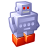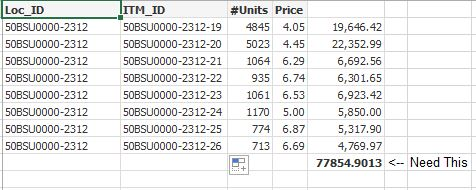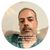Announcements
Product Release Webinar: Qlik Insider airing December 6! REGISTER TODAY!
cancel
Showing results for
Did you mean:Anonymous
Not applicable

## Aggr function in dimensionless bar chart

Hello QlikView experts,

I am trying to create a bar chart with expression total and for that I am using Aggr function. Below is my code :-

SUM(

AGGR(

(SUM([Price] *

sum({\$ <Year = {"\$(=(max(Year)-1))"},

Month = {"\$(=(month(today())))"}>}

#Units)

)

) , ITM_ID) )

Here I am multiplying Price by Units at the ITM_ID level and want to sum this on Location_ID Level. Location can be added as a dimension to the bar chart. I tried putting Location_ID in above expression but that did not help either.

For some reason it is giving me 0 in text object and is not showing up in the bar chart.

Would like to know any obvious suggestions/error just looking at the above expression?

Thanks

1 Solution

Accepted SolutionsSpecialist III

is there a specific reason why you are not multiplying price by units ?

you could use total

sum(total <[Location_ID>{\$ <Year = {"\$(=(max(Year)-1))"},

Month = {"\$(=(month(today())))"}>}

#Units*[Price])

9 RepliesMVP

AGGR function will work only on the dimension ...you need to have dimension without that it won't workSpecialist III

is there a specific reason why you are not multiplying price by units ?

you could use total

sum(total <[Location_ID>{\$ <Year = {"\$(=(max(Year)-1))"},

Month = {"\$(=(month(today())))"}>}

#Units*[Price])Anonymous
Not applicable
Author

Hi Avinash, thanks for quick reply. I have added dimension in bar chart to test my expression. It does not come up with value still! In addition to this, I also tried to add the dimension in aggr expression, see below. No luck!

=

SUM(

AGGR(

(SUM([Price] *

sum({\$ <Year = {"\$(=(max(Year)-1))"},

Month = {"\$(=(month(today())))"}>}

#Units)

)

) , ITM_ID,LOC_ID) )

What am I missing?Anonymous
Not applicable
Author

Hi Ramon, your expression is giving me some value so that is good and thanks for that! However it is different from what I am after. I am looking for sum product. Each unit value multiplied by price for that item will give me a value and I want to sum them up (for entire location.)

I have attached the spreadsheet to illustrate my requirement. Hope it is clear.Specialist III

ok, so I assume price for the itm_id can be more than once

you could try by using two aggr

 aggr(

SUM(

AGGR(

(SUM([Price] *

sum({\$ <Year = {"\$(=(max(Year)-1))"},

Month = {"\$(=(month(today())))"}>}

#Units)

)

), ITM_ID)),LOC_ID)

or by using the total outside of the aggr

SUM(total <LOC_ID>

AGGR(

(SUM([Price] *

sum({\$ <Year = {"\$(=(max(Year)-1))"},

Month = {"\$(=(month(today())))"}>}

#Units)

)

), ITM_ID))Partner - Creator III

Hi,

look at the attached sample.

Is this what you are looking for?

PatricMVP

I think direct Unit and Price multiplication should work if you take Location as Dimension in Bar chart. See the attached based on your excel sheet. Perhaps it cannot be that simple, may be missing the point you want to explainAnonymous
Not applicable
Author

Thanks for putting up a quick QVW Patric! Appreciate it. I see this is the same expression that Ramon shared and this is working for me as of now.Anonymous
Not applicable
Author

Thanks Digvijay. Yes you got it right. Just that in my case the units will actually be an aggregate of each item id (because there can be more than one item id in the table). I have used expression shared by Ramon.Community Browser